American Journal of Applied Mathematics
Volume 4, Issue 2, April 2016, Pages: 62-74

Hydrodynamic Radiating Fluid Flow Past an Infinite Vertical Porous Plate in Presence of Chemical Reaction and Induced Magnetic Field

Kibet Kiprop*, Mathew Ngugi Kinyanjui, Jackson Kioko Kwanza

Department Pure and Applied Mathematics, Jomo Kenyatta University of Agriculture and Technology, Nairobi, Kenya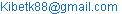(K. Kiprop)

Kibet Kiprop, Mathew Ngugi Kinyanjui, Jackson Kioko Kwanza. Hydrodynamic Radiating Fluid Flow Past an Infinite Vertical Porous Plate in Presence of Chemical Reaction and Induced Magnetic Field. American Journal of Applied Mathematics. Vol. 4, No. 2, 2016, pp. 62-74. doi: 10.11648/j.ajam.20160402.11

Abstract: An investigation of the unsteady magnetohydrodynamic fluid flow with heat and mass transfer of a viscous, incompressible, electrically conducting and Newtonian fluid past a vertical plate embedded in a porous medium taking into account induced magnetic field, first order chemical reaction and thermal radiation effect is carried out. The dimensionless governing coupled, non-linear boundary layer partial differential equations are solved by an efficient and unconditionally stable finite difference scheme of the Crank-Nicholson type. A computer software is used to iteratively solve the partial differential equations. The numerical solutions for fluid velocity, induced magnetic field, species concentration and fluid temperatures are depicted graphically. The effect of various non-dimensional parameters on the fluid flow profiles are discussed and physical interpretation given. Applications of the study include laminar magneto aerodynamics, materials processing and MHD propulsion thermo-fluid dynamics.

Keywords: Magnetohydrodynamic (MHD), Injection, Vertical Plate, Heat and Mass Transfer

1. Introduction

A fluid is a substance that continually flows under applied shear stress, regardless of its magnitude. Magnetohydrodynamic (MHD) is the study of interaction between the flow of an electrically conducting fluids and magnetic fields. The MHD problem is of great interest in current trends in mathematical modelling. This is due to its many applications in engineering problems e.g. MHD generators, plasma studies. The flow past a vertical plate has been studied by various researchers due to varied applications in MHD generators, plasma studies etc.

The study of heat and mass transfer with chemical reaction is of great practical importance to engineers and scientists because of its almost universal occurrence in many branches of science and engineering. Possible applications of this type of flow can be found in many industries like power industry and chemical process industries.
The effects of mass transfer on flow past an impulsively started infinite vertical plate with constant heat flux and chemical reaction have been studied by Das et al. . Kandasamy et al.  presented an approximate numerical solution of chemical reaction, heat and mass transfer on MHD flow over a vertical stretching surface with heat source and thermal stratification effects and effects of chemical reaction, heat and mass transfer along a wedge with heat source and concentration in the presence of suction or injection. Muthucumaraswamy and Valliammal  have presented the theoretical study of unsteady flow past an exponentially accelerated infinite isothermal vertical plate with variable mass diffusion in the presence of homogeneous chemical reaction of first order. Sharma et al.  have investigated the influence of chemical reaction and radiation on an unsteady magnetohydrodynamic free convective flow and mass transfer through viscous incompressible fluid past a heated vertical porous plate immersed in porous medium in the presence of uniform transverse magnetic field, oscillating free stream and heat source when viscous dissipation effect is also taken into account. A finite difference solution of mass transfer effects on MHD flow of incompressible viscous dissipative fluid past an exponentially accelerated isothermal vertical plate, on taking into account the viscous dissipative heat, under the influence of chemical reaction through porous medium is evaluated by Gireesh et al. .

Formulation

The two-dimensional unsteady magnetohydrodynamic mixed convective heat and mass transfer flow of a Newtonian, electrically-conducting and viscous incompressible fluid over a porous vertical infinite plate with induced magnetic field and conduction-radiation has been considered in Figure below. The vertical plate is permeable to allow for possible blowing or suction, and is infinite in the x-axis direction which is the direction of fluid flow and y-axis is normal to it. Let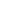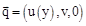be the fluid velocity and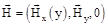be the magnetic induction vector at a point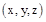in the fluid. The x -axis is taken along the plate in the upward direction, y -axis is normal to the plate into the fluid region. Since the plate is infinite in length in x -direction, therefore all the physical quantities except possibly the pressure are assumed to be independent of x. Injection of the fluid takes place through the porous wall of the vertical plate with uniform velocity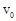which is greater than zero for injection. It is assumed that no polarization voltages exists since the plate is insulated. The wall is maintained at constant temperatureand concentration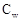higher than the ambient temperature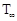and concentration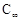respectively. The plate is non-conducting and the applied magnetic field is of uniform strength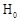and applied transversely to the direction of the main stream taking into account the induced magnetic field;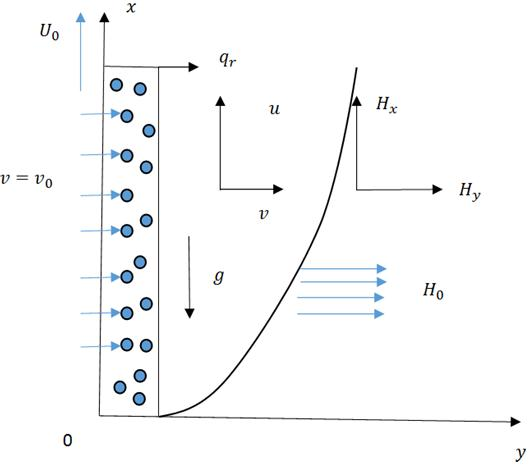Figure 1. Flow configuration.

The following assumptions are implicit in our analysis:

1. The fluid is assumed to be incompressible hence density of fluid is assumed to be constant of both time and space.

2. The ratio of the square of fluid velocity V and that of the square of velocity of light c is negligibly small

3. There is chemical reaction taking place in the fluid.

4. The plate is non-conducting.

5. The magnetic field is considered relatively strong and constant.

6. A short circuit problem where no charge accumulate is considered. Thus electrostatic force is negligible.

7. Fluid is Newtonian.

8. Fluid has constant thermal conductivity.

9. Fluid flow is assumed to be laminar.

1.1. Governing Equations

1.1.1. Equation of Continuity

The equation of continuity also known as equation of conservation of mass is derived based on two fundamental principles namely; The mass of the fluid remains constant as the fluid particles flow in the flow field. The continuum hypothesis which states that there are empty spaces between particles that were in contact and that the fluid volume is not affected by an increase in pressure i.e. the flow is continuous.

The equation can be expressed in tensor form as Hughes et al. (1964)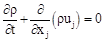(1)

By the assumption that the fluid if incompressible the equation (1) reduces to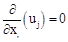(2)

1.1.2. Momentum Equation

The equation of conservation of momentum is derived from the Newton’s second law of motion, which states that the time rate change of momentum of a body matter is equal to the net external forces applied to the body. Using the fact that the flow is incompressible with invariant viscosity the equation becomes;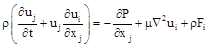(3)

Since there is no large velocity gradient here, the viscous term in Equation (3) vanishes for small μ and hence for the outer flow, beside there is no induced magnetic field along x-direction gradient, so we have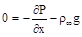(4)

The Boussinesq Approximation gives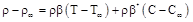(5)

From equation of continuity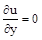and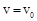since velocity in y- direction is injection velocity. We regard the porous medium as an assembly of small identical spherical particles fixed in space, considering Brinkman (1947) for porous medium. From equations above momentum equation reduces to;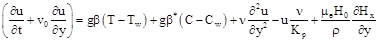(6)

1.1.3. Energy Equation

The equation of conservation of energy is derived from the First Law of Thermodynamics, which states that the amount of heat added to a system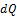equals change in internal energy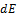plus the work done. It can be expressed as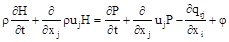(7)

In this study we consider the flow of viscous incompressible fluid with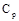being a constant, we consider radiation and viscous dissipation, and then the energy equation can be expressed as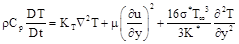(8)

1.1.4. Concentration Equation

The equation of species concentration is based on the law of conservation of mass. This equation is used when the porous medium is saturated with fluid and obeys Darcy’s law. Convection is one of the major modes of heat transfer and mass transfer. The equation of species concentration is given as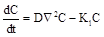(9)

From equation (9) we can come up with the following equation;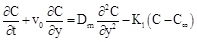(10)

1.1.5. Induction Equation

Since the magnetic field is varied we have to solve the magnetic induction equation given by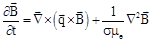(11)

It describes the evolution of magnetic field. The first time on the right-hand side is the advective term that describes the interaction of the field with the flow velocity. The second term on the right hand side is a diffusive term. In the absence of the fluid flow velocity, the diffusive term leads to a decay of magnetic field. The magnetic induction equations is given as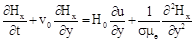(12)

The equations (7), (8), (10) and (12) are the specific equations for the problem and are solve simultaneously. Subject to initial and boundary conditions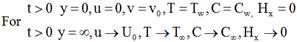(13)

1.2. Non-dimensionalization

Non-dimensionalization has the ability to reduce complicated physical problem into more feasible one for solutions and also enable scientists work with model without limiting themselves to specific measurements and units. Theoretical solutions obtained in non-dimensional form are free of units and more flexible. In the present MHD problem we are define the various non-dimensional parameters and then deal with the non-dimensionalization process.

1.2.1. Non-dimensional Parameters

The following non-dimensional parameters were considered in this paper.

1.2.2. Prandtl Number, Pr

This is the ratio of fluid properties controlling the velocity and the temperature distributions. It is the ratio of viscous force to thermal force.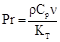(14)

1.2.3. Local Temperature Grashof Number, Gr

This is a non-dimensional number which normally occurs in natural convection problem. It is the ratio of thermal buoyancy forces to viscous hydrodynamic forces.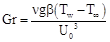(15)

1.2.4. Local Mass Grashof Number, Grm

It is the ratio of species buoyancy forces to viscous hydrodynamic forces.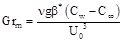(16)

1.2.5. Eckert Number, Ec

This expresses the relationship between the kinetic energy in the flow and the enthalpy. It represents the conversion of kinetic energy into internal energy by work that is done against the viscous fluid stresses.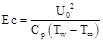(17)

1.2.6. Schmidt Number, Sc

The Schmidt number quantifies the relative effectiveness of momentum and mass transport by diffusion in the hydrodynamic (velocity) and the concentration (species) boundary layers. It physically relates the relative thickness of the hydrodynamic layer and mass transfer boundary layer.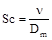(18)

1.2.7. Hartmann Number

This is the ratio of magnetic force to viscous force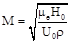(19)

1.2.8. Magnetic Prandtl Number

This approximates ratio of momentum diffusivity and magnetic diffusivity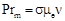(20)

1.2.9. Permeability Parameter

Measures effective permeability of the phase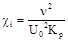(21)

1.3. Non-dimensionalizing Equations Governing Fluid Flow

For this particular problem we let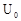to be characteristic velocity in freestream. To non-dimensionalize the equations governing the flow we used the transformations: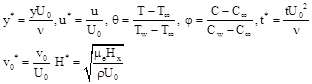(22)

Using the transformations (22) and with help of (21), (20), (19), (18), (16), (15) and (14) the non-dimensional forms of (6), (8), (10) and (12) are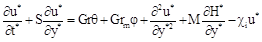(23)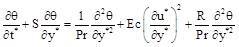(24)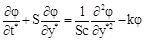(25)(26)

The corresponding boundary conditions are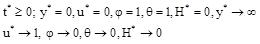(27)

2. Method of Solution

The final set of governing equations (21), (22), (23) and (24) cannot be solved numerically since they are highly coupled and nonlinear. Together with the boundary conditions (25), a numerical solution is constructed using finite difference method (FDM). The velocity, temperature, concentration and induction are functions of space (y) and time (t). There is therefore a necessity to discretize the time and space coordinates to form a solution mesh.

Using Crank-Nicolson method the momentum equation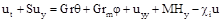(28)

Becomes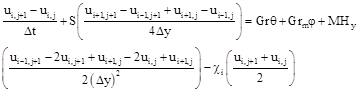(29)

From equation (29) the values of u at time step j+1 and j are appearing on both sides of the equation. This equation (29) is used to predict the values of u at time step j+1, so all values of u at time step j are assumed to be known. We rearrange equation (29) above so that values of u at time j+1 are on the left and values of u at time j so that we get;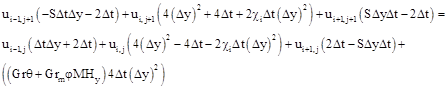(30)

The Crank-Nicolson scheme is implicit, hence a system of equations for u must be solved at each time step.

If we let coefficients of interior nodes be;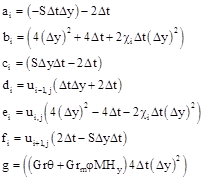(31)

For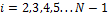Substituting equations from (31) into equation (30) we get;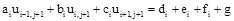(32)

The system of equations can be represented in matrix for as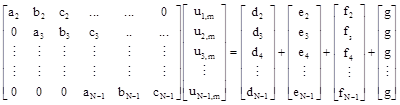(33)

Doing the same for Energy Equation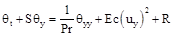(34)

In matrix form we can represent this as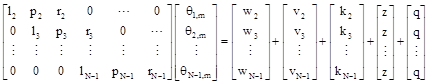(35)

For the concentration equation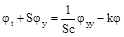(36)

In matrix form we can represent this as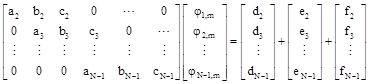(37)

The Induction equation is written as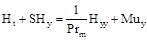(38)

In matrix form we can represent this as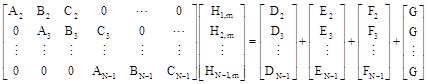(39)

Finally, the block of tridiagonal matrices (33), (35), (37) and (39) are solved using MATLAB algorithm and results presented in graph which we are going to do in the next chapter.

3. Results and Discussion

Since the problem has been non-dimensionalized, the default values are chosen which are used to determine the changes which will bring to the fluid flow. The choice made for default values of fluid properties are;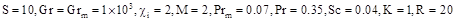(40)

3.1. Effects of Magnetic Parameter

While keeping all fluid properties at their default values, the magnetic parameter was varied as M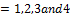. The results obtained are presented in figure below.

It is observed that magnetic parameter affects both velocity and induced magnetic field. The magnetic parameter increases with decrease in both velocity and induced magnetic field. The above observations is as a result of the final set of governing equations. It can be deduced that the velocity of the fluid decreases with increase in magnetic parameter. We also notice that there is a fall in induced magnetic field as magnetic parameter increases. It is noticed that induced magnetic flux peaks a short distance from the plate, and then decays to zero in free stream.

Physically, the magnetic parameter is a measure of the magnetic field strength within the fluid. It actually represents ratio of electromagnetic forces to viscous forces. The presence of a magnetic field in an electrically conducting fluid introduces a force called Lorentz force, which acts against the fluid if the magnetic field is applied in the normal direction.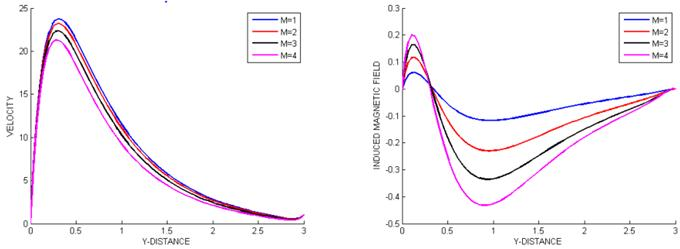Figure 2. Fluid flow profiles for various magnetic parameters.

3.2. Effects of Injection Velocity

While keeping all fluid properties at their default values, the Injection parameter was varied S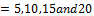. The results obtained are presented in figure below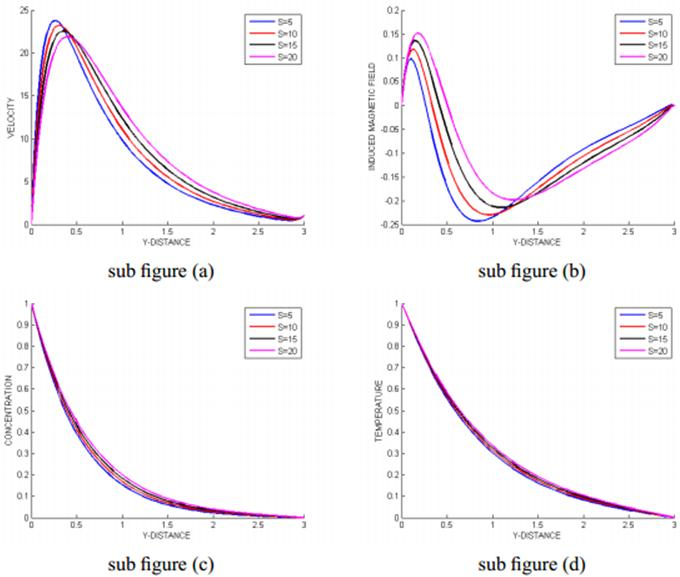Figure 3. Fluid flow properties for various injection parameter.

It is observed that injection velocity has a significant effect on velocity, temperature, concentration and induced magnetic field profiles. An increase in injection parameter leads to increase in velocity, temperature, concentration and induced magnetic field. Velocity near the plate increases owing to presence of foreign gases in flow field.

Increase in injection causes a rise in concentration profiles. Injection means and increase in molecular diffusivity which consequently result in the rise of concentration.

3.3. Effects of Prandtl Number

While maintaining the other fluid properties at their default values, the Prandtl number was varied Pr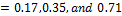. The results obtained are shown in the figure below.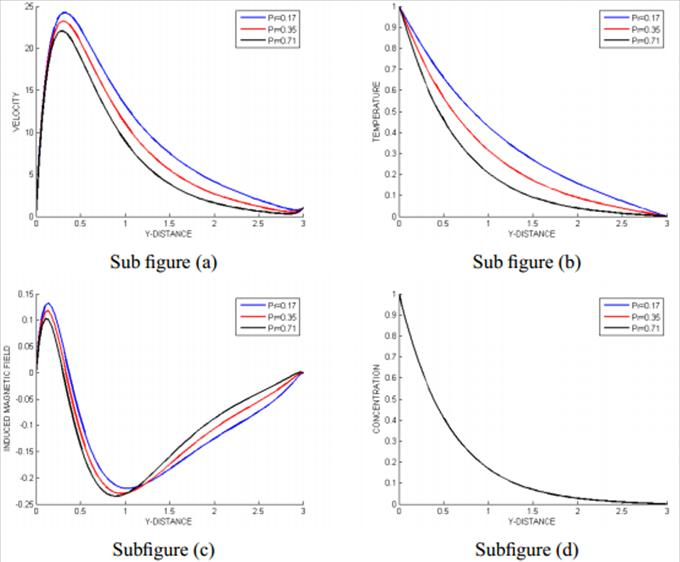Figure 4. Fluid flow properties for various Prandtl Number.

It is observed that increase in Prandtl number leads to decrease in velocity, temperature and induced magnetic field. Except for small region near the plate. No effects on the concentration.

Physically, increase in Prandtl number leads to decrease in thermal boundary layer and lower average temperature within boundary layer. The smaller Prandtl number is equivalent to increase in thermal conductivity of fluid and heat is able to diffuse away from heated surface more rapidly for higher values of Prandtl number. As a result the flow is decelerated. Higher Prandtl number possess higher viscosities implying that such fluids will flow slower than lower Prandtl fluids. As a result velocity will decrease substantially with increase in Prandtl number.

We notice that induced magnetic field decrease with increase in Prandtl number and also magnetic parameter.

3.4. Effects of Eckert Number

While maintaining the other fluid properties at their default values, the Eckert number was varied as Ec = 0.002, 0.01, 0.03and 0.05. The results obtained are shown in the figure below.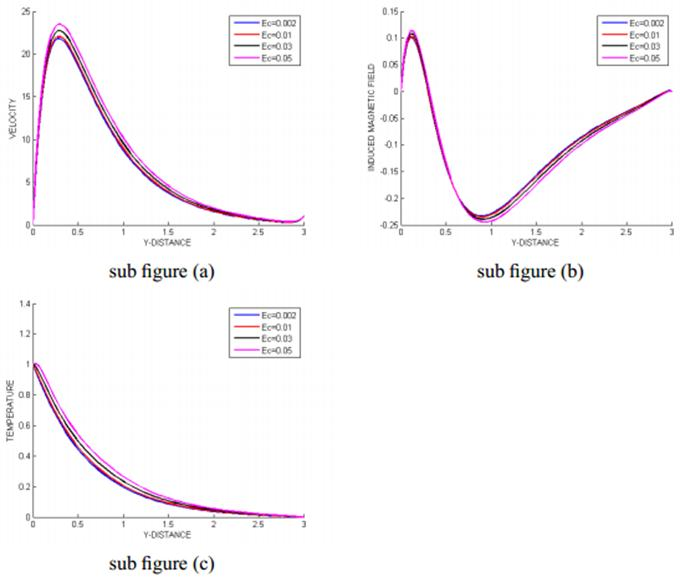Figure 5. Fluid flow properties for various Eckert Numbers.

From the graphs we can observe that Eckert number has significant effect on temperature, velocity and induced magnetic field. The Eckert number affects the temperature profiles the most because from the governing equations it directly affect the energy equation. We can observe from the graphs that as Eckert number is increased the temperature of the fluid flow increases hence increases the velocity of the fluid flow. Eckert number has no much effect on induced magnetic field near the plate but at free it reduces. Physically, Eckert is the ratio of kinetic energy to the enthalpy. This means that a larger Eckert number implies more kinetic energy. The effects of viscous dissipation on the flow field is to increase the energy, yielding a greater temperature and as a consequence greater buoyancy force. This enhance the temperature hence increase in velocity of fluid flow.

3.5. Effects of Schmidt Number

While maintaining the other fluid properties at their default values, the Schmidt number was varied as Sc = 0.02, 0.04, 0.1and 0.2. The results obtained are shown in the figure below

We observe that Schmidt number affects mostly the velocity, induced magnetic field and concentration profiles. There is no observable effects on temperature profiles. Increase in Schmidt number leads to reduction in velocity and concentration profiles and also reduction of induced magnetic field near the plate and increase in the free stream. Mathematically, these observations can be explained from the relation in the governing equations above. We notice that there exists an inverse relationship between the concentration and Schmidt number, therefore the inhibition of concentration profile on increasing Schmidt number. The effects on velocity and induced magnetic field is affected by the concentration.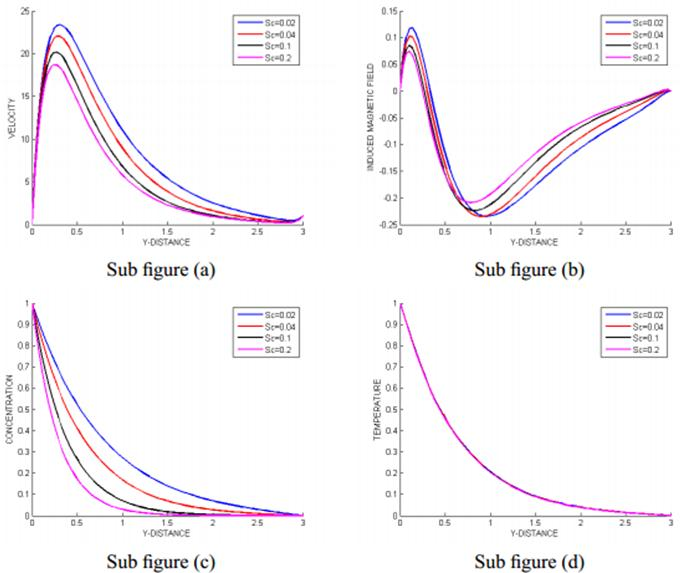Figure 6. Fluid flow properties for various Schmidt number.

3.6. Effects of Grashof Parameters

While maintaining the other fluid properties at their default values, the Grashof parameter was varied as Gr = 1× 101, 1× 102 and 1× 103. The results obtained are shown in the figure below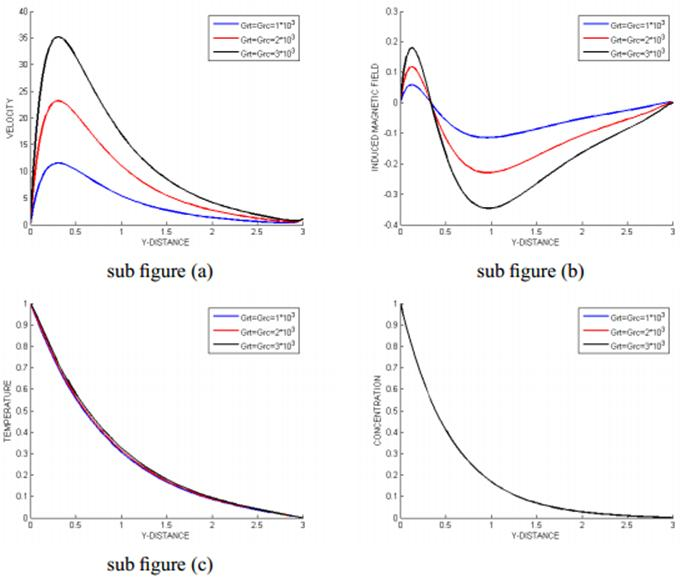Figure 7. Fluid profile for various Grashof numbers.

We observe from the graphs some significant change in velocity, temperature and induced magnetic field but no much change in concentration. We notice that when Grashof Number is increased the velocity and the temperature of fluid flow increases. For the induced magnetic field it increases near the plate and decays to the relevant free stream.

Physically, increase in Grashof number leads to an upward acceleration of fluid in the vicinity of vertical wall caused by increasing buoyancy. A rise in velocity and temperature means heating of fluid. Curves shows that the peak values of velocity increase rapidly near the wall of the porous plate as Grashof number increases and decays to the relevant free stream velocity. A positive increase in Grashof clearly accelerates velocities. Grashof number is proportional to the thermal buoyancy generated by free convection currents. Increase in buoyancy will therefore aid the flow.

While maintaining the other fluid properties at their default values, the Radiating parameter was varied as R=10,20and30. The results obtained are shown in the figure below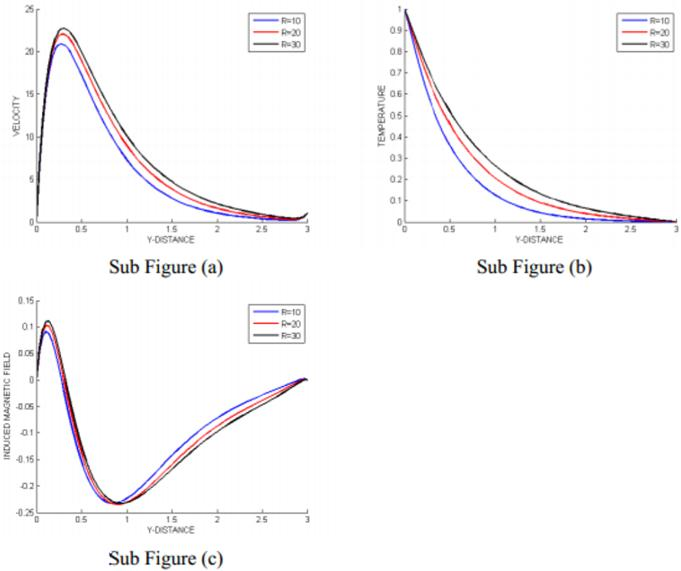Figure 8. Fluid flow profile for various radiating parameter.

We can observe that from the graphs that as we increase Radiating parameter the velocity, temperature and induced magnetic field increases.

Physically, a large value of radiating parameter corresponds to an increased dominance of thermal radiation over conduction. Thermal radiation supplements thermal diffusion and increases the overall thermal diffusivity of the regime. Since he local radiant diffusion flux model adds radiation conductivity to the convectional thermal conductivity. As a result the temperature in the fluid region is significantly increased hence increased velocity of the fluid flow.

3.8. Effects of Permeability Parameter

While maintaining the other fluid properties at their default values, the Permeability parameter was varied as χ i =1, 2, 4. The results obtained are shown in the figure below.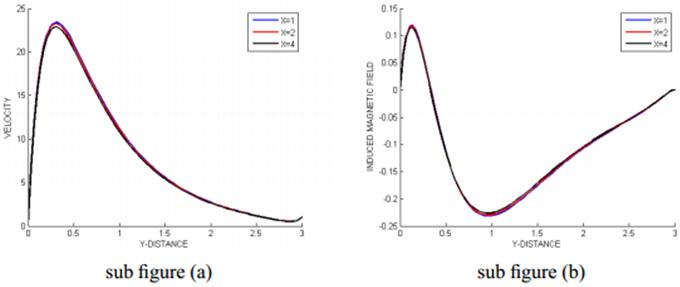Figure 9. Fluid flow for various permeability parameter.

9 Flow profile for various Permeability parameter values.

We observe some slight changes in the graphs as permeability parameter is changed. We can observe that as permeability parameter is increased the velocity of fluid reduces slightly. And no much significant change in induced magnetic field as it has small change near the plate and increases in freestream slightly.

Physically, presence of porous medium increases resistance to flow resulting in decrease in flow velocity.

4. Conclusion

In this paper effects of chemical reaction and viscous dissipation on MHD free convection flow past a porous vertical plate with variable surface temperature and concentration have been studied numerically. Implicit finite difference method is employed to solve the equations governing the flow. From the present numerical investigation. The study concluded that visco-elasticity decreased the temperature profiles in the flow field for small values of the Prandtl number; and that the temperature profiles decreased with increase in the strength of the magnetic field. From the present numerical investigation, following conclusions have been drawn:

It is found that the velocity decreases with increasing magnetic parameter (M).

Under the influence of chemical reaction, the flow velocity reduces

The increasing value of the viscous dissipation parameter enhancing the flow temperature

A decrease in concentration with increasing Schmidt number as well as chemical reaction parameter is observed.

It is marked that the rate of concentration transfer increases with increasing values of chemical reaction parameter ‘K’ and Schmidt number ‘Sc’.

Nomenclature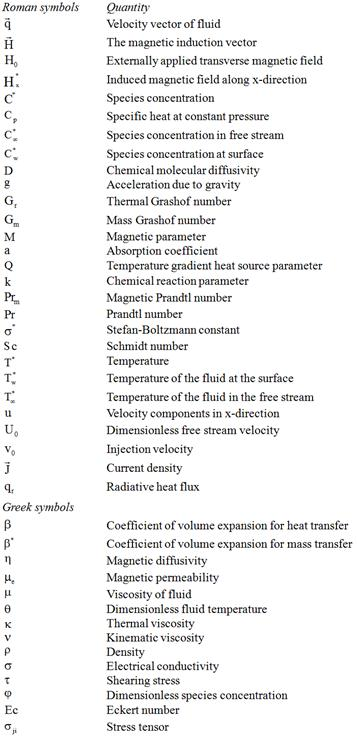Acknowledgement

I thank my parents and my supervisors for the support throughout my master’s program.

References

1. Huges, W.F., Yong, F.J. The electro-Magneto-Dynamics of fluids, John Wiley & Sons, New york, USA, 1966.
2. Sacheti, N.C., Chandraan, P., Singh, A.K. Int. Comm., Heat Mass Transfer, 21,1,131–142, 1994.
3. Takar, H.S., Gorla, R.S.R. and Soundalgekar, V.M. Int. J. Numerical Methods for Heat & Fluid Flow, 6, 77–83, 1996.
4. Abd-El-Naby M.A., Elasayed M.E., Elbarbary Nader Y. and Abdelzem. J.Appl.Math, 2, 65–86, 2003.
5. Ramachandra Prasad, V., Bhaskar Reddy, N. and Muthucumaraswamy, R. J. Appl. Theo. Mech., 33, 31–63, 2006.
6. Samaria, N.K., Reddy, M.U.S., Prasad, R. and Gupta. H.N. Springer Link, 179, 1, 2004.
7. Sreehari Reddy, P., Nagarajan, A.S. and Sivaiah, M. Journal of Naval Architecture and Marine Engng, 2, 47–56, 2008.
8. Israel – cookey, C., Ogulu, A., Omubo – Pepple, V.M.,Int. J. Heat Mass Transfer, 46, 13, 2305–2311, 2003.
9. Zueco Jordan, J., Appl.Math.Modeling, 31, 20, 2019–2033, 2007.
10. Suneetha, S., Bhaskar Reddy, N., Ramachandra Prasad, V. Thermal Science, 13, 2, 71–181, 2009.
11. Hitesh Kumar, Thermal Science 13, 2, 163–169, 2009.
12. U.N. Das, R. Deka, V.M. Soundalgekar, Forsch. Ingenieurwes., 60, pp. 284–287, 1994.
13. R. Kandasamy, K. Periasamy, K.K.S. Prabhu. Int. J.Heat Mass Transfer, V48, pp. 4557-4561, 2005.
14. R. Muthucumaraswamy, V.Valliammal, Theoret. Appl. Mech., Vol.37, No.4, pp. 251–262, Belgrade 2010.
15. P. R. Sharma, Navin Kumar and Pooja Sharma, Applied Mathematical Sciences, Vol. 5, No.46, 2249–2260, 2011.
16. Girish Kumar, J., Kishore, P. M., Ramakrishna, S. Advances in Applied Science Research, 2012, 3 (4), 2134-2140.
17. P.M. Kishore, V. Rajesh, S. Vijaya Kumar Verma, Journal of Naval Architecture and Marine Engineering, 7, 101–110, 2010.
18. Brewster, M.Q. Thermal Radiative Transfer and Properties, John Wiley & Sons, New York, USA, (1992).
19. Carnahan, B., H.A. Luther, J.O. Wilkes, Applied Numerical Methods, John Wiley &Sons, New York, (1969).

 Contents 1. 1.1. 1.2. 1.3. 2. 3. 3.1. 3.2. 3.3. 3.4. 3.5. 3.6. 3.7. 3.8. 4.
Article ToolsAbstractPDF(2636K)